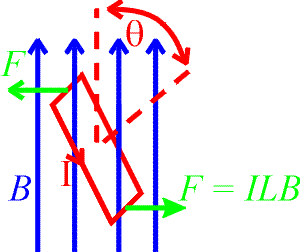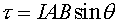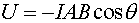Magnetic dipoles

This topic was not covered (Spring 1999, Spring 2000)

A magnetic dipole is simply a current loop. A current loop both creates and responds to magnetic fields. First let's review the torque felt by a dipole in a magnetic field. Consider the current loop in a magnetic filed below. The two forces are equal and opposite and do not act to move the dipole, only to rotate it.The moment arm for the torque is the height of the dipole, h sinq. the angle q is between the direction of the magnetic field and a vector normal to the plane of the dipole. Therefore the torque disappears when the dipoles orientation is along the axis of the magnetic field (the dipole lies in a horizontal plane in the picture above.) The torque always tries to rotate the angle q to zero. This is where the potential energy is a minimum. The torque and potential energy are:where A is the area of the dipole's loop. The product IA is refered to as the dipole moment.

Examples       Magnetic field sources index        Lecture index